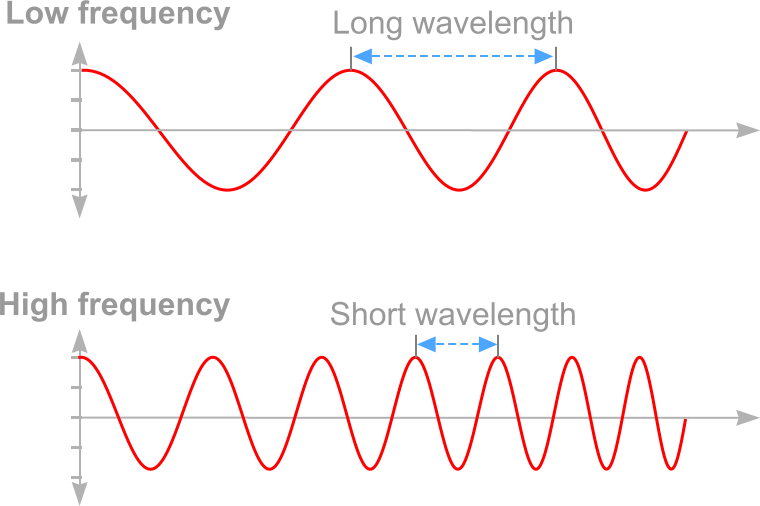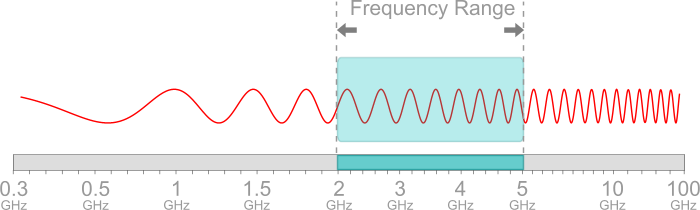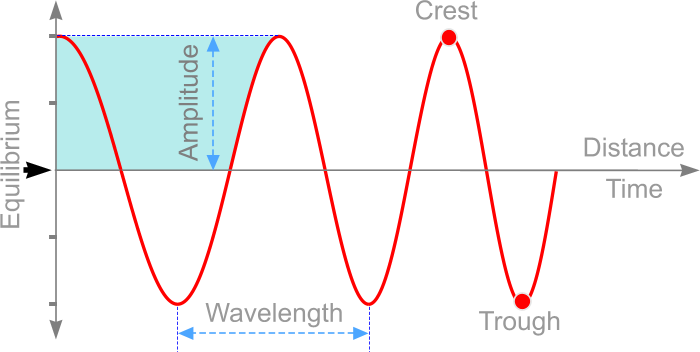## EMF Explained

Electric and magnetic fields (EMF) are an invisible force that surrounds any electrical device.
Electrical equipment like mobile phones, Wi-Fi routers, cell towers, power lines, electrical wiring, all produces EMF.

EM waves are classified according to their frequency: Rradio waves, microwaves, infrared waves, visible light waves, ultraviolet waves, x-rays and gamma rays.

Let's take a closer look, shall we?

The rate at which something occurs over a particular period of time. In the context of our fabrics, frequency describes the number of waves that pass a fixed point in a given amount of time.For example if the time it takes for a wave to pass is ½ second, the frequency is 2 per second. If it takes 1/100 of an hour, the frequency is 100 per hour.

Frequency is measured in Hertz (Hz) and is named after the German physicist Heinrich Hertz.
Frequency is the measure of 1 wave cycle per secod (1 Hz).

Units of measure

 1 Hz = 1 cycle per second 1000 Hz = 1 Kilohertz (kHz) 1000 kHz = 1 Megahertz (MHz) 1000 MHz = 1 Gigahertz (GHz) 1 GHz  =  1 Billion ( 1,000,000,000 Hz )

Is a range of fequencies of relevance in the electromagnetic spectrum.

For example:
If a freqency range of 2 GHz to 5 GHz is stated, this means frequencies from 2 GHz to 5 GHz is of interest.Amplitude, in our case, is the maximum distance moved by a point on a wave measured from its equilibrium position.
As in sound waves, amplitude is the measure of displacement of the wave (loudness) from its rest position (silence).

The amplitude is shown on the graph below.By definition, it means 'to diminish'.

An example of attenuation is when earplugs are worn to sleep, where the volume of sound around is greatly reduced when passing through the foam material.

When a signal travels through a medium, its energy is diminished, resulting in a lower amplitude of the wave.

This loss in amplitude is what is defined as attenuation and is measured in decibels.

A decibel (dBs) is a measure of the increase or decrease in the amount of power between two points A and B (see diagram).

As electromagnetic frequencies pass through bloqed clothing and apparels, the energy is greatly reduced or attenuated.

A rainbow in the sky, watching TV, remote control for your garage door or using a cell phone, what they all have in common, is that they all involve the electromagnetic spectrum.

Electromagnetic waves are a form of energy that has both an electric and magnetic field and wireless technologies use a frequency range of the electromagnetic spectrum.

Here is a snapshot of what goes on inside the part of the electromagnetic spectrum we use.

Note: 5G uses a much higher frequency range. The chart above has been adjusted visually, compressing the higher frequencies into a shorter spacing.

Another way to visualise the higher frequencies is in a linear chart (as below) where the GHz units are evenly spaced.
What has become common is the frequency range between 2.4 GHz to 6 GHz and what has been outlined are the frequencies addressed by Bloqed fabrics with a frequency range from 0.9 GHz to 10 GHz.